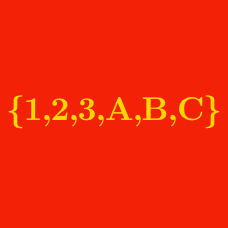Discrete Mathematics

Sets - Definition of terminology

If set $S$ has $|S|=3$ elements and satisfies \begin{aligned} \text{(i)} &\; 0 \in S \text{ and} \\ \text{(ii)} &\; \text{if } p \in S, q \in S \text{ and } p \neq q, \text{ then } (p+q) \in S \end{aligned} and $10$ is an element of $S,$ what is the other element of $S$ excluding $0$ and $10?$

Note: You may choose to read the summary page Set Notation.

If $A$ is the set of all odd positive integers less than $50,$ what is the number of elements in $A?$

If $A$ is the set of all integers satisfying $11 \leq x \leq 27,$ what is the number of elements in $A?$

If $A$ is the set

$A= \{13, 17, 9, 0, -13\},$

what is $\lvert A \rvert?$

Details and assumptions

You may choose to read the summary page Set Notation.

How many elements are in the set $\{10, 12, 14, \ldots, 48, 50\}$?

×